/

### The Chi-squared distribution

The chi-squared distribution with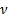degrees of freedom is the distribution of a sum of the squares ofindependent standard normal random variables. A consequence is that the sum of independent chi-squared variables is also chi-squared distributed. It is widely used in hypothesis testing, goodness of fit analysis or in constructing confidence intervals. It is a special case of the gamma distribution.

 Distribution name Chi-squared distribution Common notation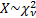Parameters= degrees of freedom (positive integer) Domain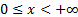Probability density function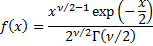Cumulative distribution function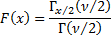MeanVariance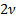Skewness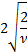(Excess) kurtosis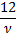Characteristic function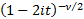Other comments Its median is approximately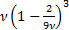. Its mode is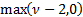. Is also known as the central chi-squared distribution (when there is a need to contrast it with the noncentral chi-squared distribution).   In the special case of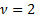the cumulative distribution function simplifies to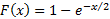.   As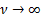,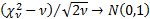and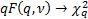Nematrian web functions

Functions relating to the above distribution may be accessed via the Nematrian web function library by using a DistributionName of “chi-squared”. Functions relating to a generalised version of this distribution including additional location (i.e. shift) and scale parameters may be accessed by using a DistributionName of “chi-squared3” ”, see also including additional shift and scale parameters. For details of other supported probability distributions see here.